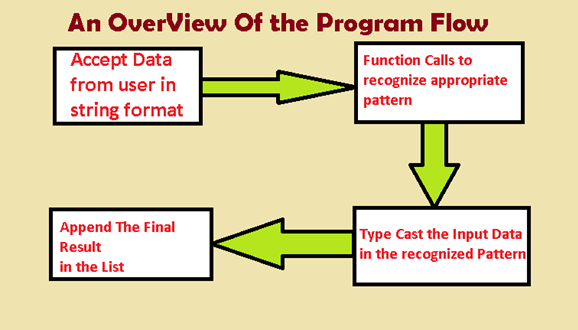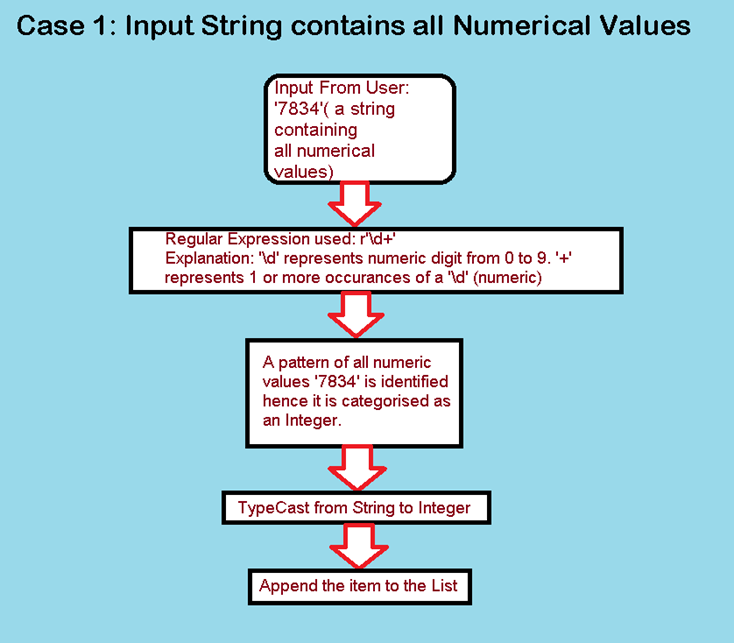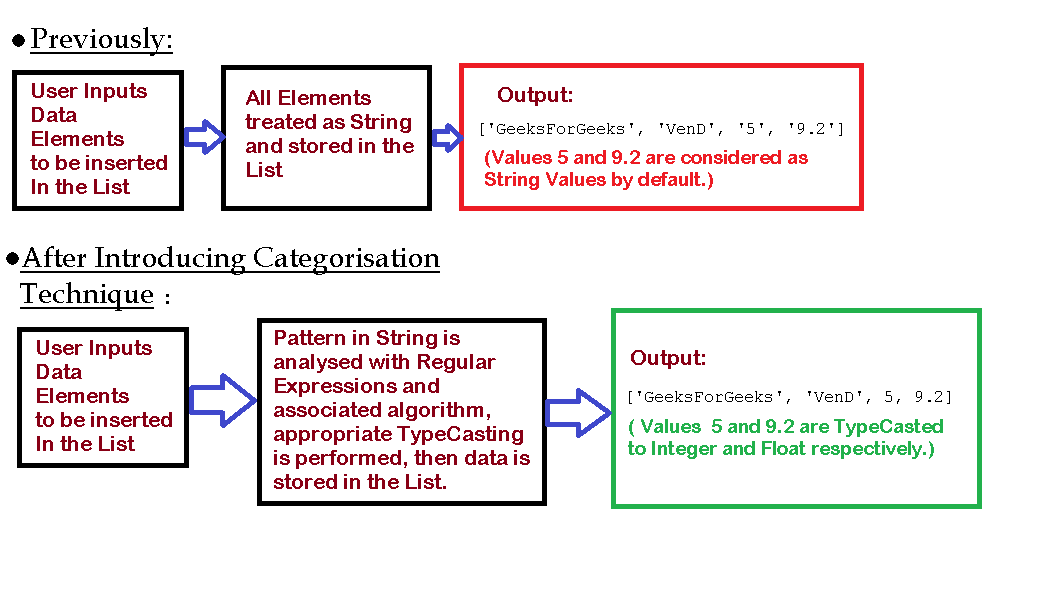# Python | Categorizing input Data in Lists

Lists in Python are linear containers used for storing data of various Data Types. The ability to store a variety of data is what makes Lists a very unique and vital Data Structure in Python.
Once created, lists can be modified further depending on one’s needs. Hence, they are ‘mutable.’

Lists, when created and by defining the values in the code-section, generates an output similar to this:
Code :

 `List` `=``[``'GeeksForGeeks'``, ``'VenD'``, ``5``, ``9.2``] ` `print``(``'\n List: '``, ``List``) `

Output:

```
List:  ['GeeksForGeeks', 'VenD', 5, 9.2]
```

In the above picture, the defined list is a combination of integer and string values. The interpreter implicitly interprets ‘GeeksForGeeks’ and ‘VenD’ as string values whereas 5 and 9.2 are interpreted as integer and float values respectively. We can perform the usual arithmetic operations on integer and float values as follows.
Code :

 `# Usual Arithmetic Operations on 5 and 9.2: ` `List` `=``[``'GeeksForGeeks'``, ``'VenD'``, ``5``, ``9.2``] ` `print``(``'\n List+2, Answer: '``, end ``=``'') ` `print``(``List``[``List``.index(``5``)]``+``2``) ` ` `  `print``(``'\n\n List+8.2, Answer: '``, end ``=``'') ` `print``(``List``[``List``.index(``9.2``)]``+``8.2``) `

Output:

``` List+2, Answer: 7
```

Also, the string-specific operations like string concatenation can be performed on the respective strings:
Code :

 `# String Concatenation Operation ` `# List:  ['GeeksForGeeks', 'VenD', 5, 9.2] ` `# Concatenating List and List ` `List` `=`  `[``'GeeksForGeeks'``, ``'VenD'``, ``5``, ``9.2``] ` `print``(``List``[``0``]``+``' '``+``List``[``1``]) `

However, since we know that lists contain items of various data types those which might be of type: string, integer, float, tuple, dictionaries, or maybe even list themselves (a list of lists), the same is not valid if you are generating a list as a result of user input. For instance, consider the example below:
Code :

 `# All Resultant Elements of List2 will be of string type ` ` `  `list2 ``=``[]  ``# This is the list which will contain elements as an input from the user ` ` `  `element_count ``=` `int``(``input``(``'\n Enter Number of Elements you wish to enter: '``)) ` ` `  `for` `i ``in` `range``(element_count): ` `    ``element ``=` `input``(f``'\n Enter Element {i + 1} : '``) ` `    ``list2.append(element) ` `     `  `print``(``"\n List 2 : "``, list2) `

Output:

```
Enter Number of Elements you wish to enter: 4

Enter Element 1 : GeeksForGeeks

Enter Element 2 : VenD

Enter Element 3 : 5

Enter Element 4 : 9.2

List 2 :  ['GeeksForGeeks', 'VenD', '5', '9.2']

```

You may notice that List2 generated as a result of User Input, now contains values of string data-type only. Also, the numerical elements have now lost the ability to undergo arithmetic operations since they are of string data-type. This behaviour straightaway contradicts the versatile behavior of Lists.

It becomes necessary for us as programmers to process the user data and store it in the appropriate format so that operations and manipulations on the target data set become efficient.
In this approach, we shall distinguish data obtained from the user into three sections, namely integer, string, and float. For this, we use a small code to carry out the respective typecasting operations.

A technique to Overcome the proposed Limitation:Code :

 `import` `re ` ` `  `def` `checkInt(string): ` `    ``string_to_integer ``=` `re.``compile``(r``'\d+'``) ` `    ``if` `len``(string_to_integer.findall(string)) !``=` `0``: ` `        ``if` `len``(string_to_integer.findall(string)[``0``])``=``=` `len``(string): ` `            ``return` `1` `        ``else``: ` `            ``return` `0` `    ``else``: ` `        ``return` `0` `     `  `def` `checkFloat(string): ` `    ``string_to_float ``=` `re.``compile``(r``'\d*.\d*'``) ` `    ``if` `len``(string_to_float.findall(string)) !``=` `0``: ` `        ``if` `len``(string_to_float.findall(string)[``0``])``=``=` `len``(string): ` `            ``return` `1` `        ``else``: ` `            ``return` `0` `    ``else``: ` `        ``return` `0` `     `  `List2 ``=``[] ` `element_count ``=` `int``(``input``(``'\n Enter number of elements : '``)) ` ` `  `for` `i ``in` `range``(element_count): ` `    ``input_element ``=` `input``(f``'\n Enter Element {i + 1}: '``) ` ` `  `    ``if` `checkInt(input_element): ` `        ``input_element ``=` `int``(input_element) ` `        ``List2.append(input_element) ` `         `  `    ``elif` `checkFloat(input_element): ` `        ``input_element ``=` `float``(input_element) ` `        ``List2.append(input_element) ` `         `  `    ``else``: ` `        ``List2.append(input_element) ` `         `  `print``(List2) `

Output:

``` Enter number of elements : 4

Enter Element 1: GeeksForGeeks

Enter Element 2: VenD

Enter Element 3: 5

Enter Element 4: 9.2
['GeeksForGeeks', 'VenD', 5, 9.2]
```

The above technique is essentially an algorithm which uses the Regular Expression library along with an algorithm to analyze the data-types of elements being inserted. After successfully analyzing the pattern of data, we then proceed to perform the type conversion.

For example, consider the following cases:

1. Case1: All values in the string are numeric. The user input is 7834, the Regular Expression function analyzes the given data and identifies that all values are digits between 0 to 9 hence the string ‘7834’ is typecasted to the equivalent integer value and then appended to the list as an integer.

Expression Used for Integer identification : r’\d+’2. Case2: The String expression contains elements which represent a floating point number. A floating point value is identified over a pattern of digits preceding or succeeding a full stop(‘.’). Ex: 567., .056, 6.7, etc.
Expression used for float value identification: r’\d*.\d*’
3. Case3: String Input contains characters, special characters and numerical values as well. In this case, the data element is generalised as a string value. No special Regular Expression needed since the given expression will return false when being categorised as Integer or Float Values.
Ex: ‘155B, Baker Street ! ‘, ’12B72C_?’, ‘I am Agent 007’, ‘_GeeksForGeeks_’, etc.

Conclusion: This method, however, is just a small prototype of typecasting values and processing raw data before storing it in the list. It definitely offers a fruitful outcome which overcomes the proposed limitations on list inputs. Further, with the advanced applications of regular expressions and some improvements in the algorithm, more forms of data such as tuples, dictionaries, etc. can be analysed and stored accordingly.• Since data is stored in the appropriate format, various ‘type-specific’ operations can be performed on it. Ex: Concatenation in case of strings, Addition, Subtraction, Multiplication in case of numerical values and various other operations on the respective data types.
• Typecasting phase takes place while storing the data. Hence, the programmer does not have to worry about Type Errors which occur while performing operations on the data set.

Limitations of Dynamic TypeCasting:

• Some data elements which do not necessarily require typecasting undergo the process, resulting in unnecessary computing.
• Multiple condition-checks and function calls result in memory wastage when each time a new element is inserted.
• The flexibility of processing numerous types of data might require several new additions to the existing code as per the developer’s needs.

Attention geek! Strengthen your foundations with the Python Programming Foundation Course and learn the basics.

To begin with, your interview preparations Enhance your Data Structures concepts with the Python DS Course.

My Personal Notes arrow_drop_upThird Year Computer Engineering Student at SPPU

If you like GeeksforGeeks and would like to contribute, you can also write an article using contribute.geeksforgeeks.org or mail your article to contribute@geeksforgeeks.org. See your article appearing on the GeeksforGeeks main page and help other Geeks.

Please Improve this article if you find anything incorrect by clicking on the "Improve Article" button below.

Article Tags :

1

Please write to us at contribute@geeksforgeeks.org to report any issue with the above content.